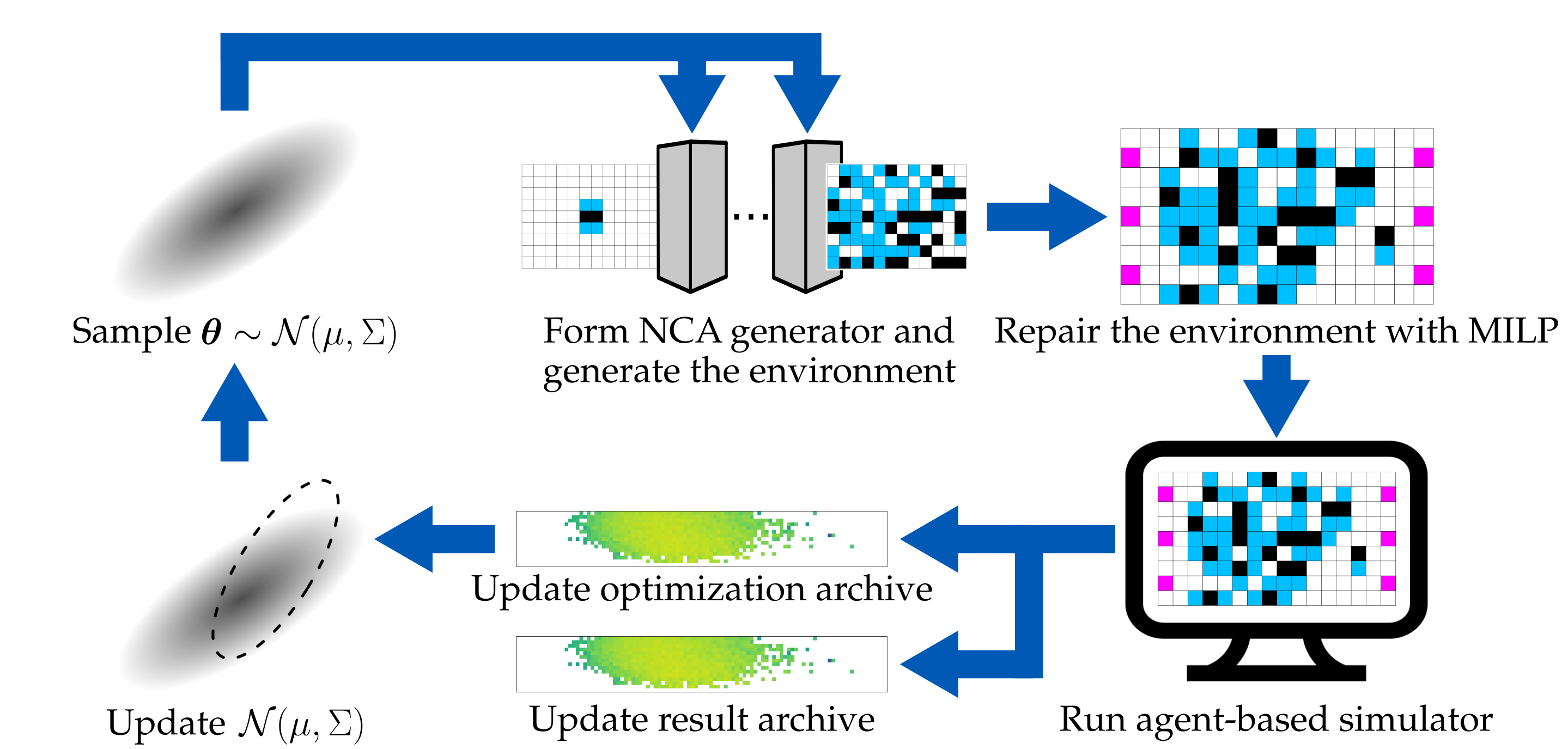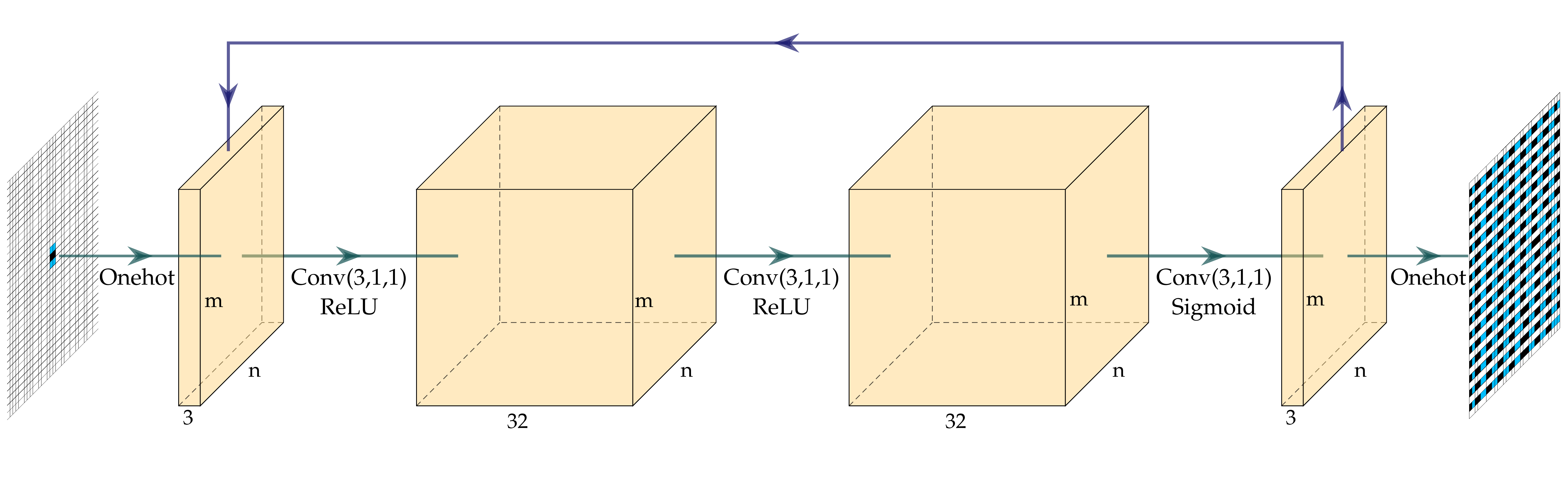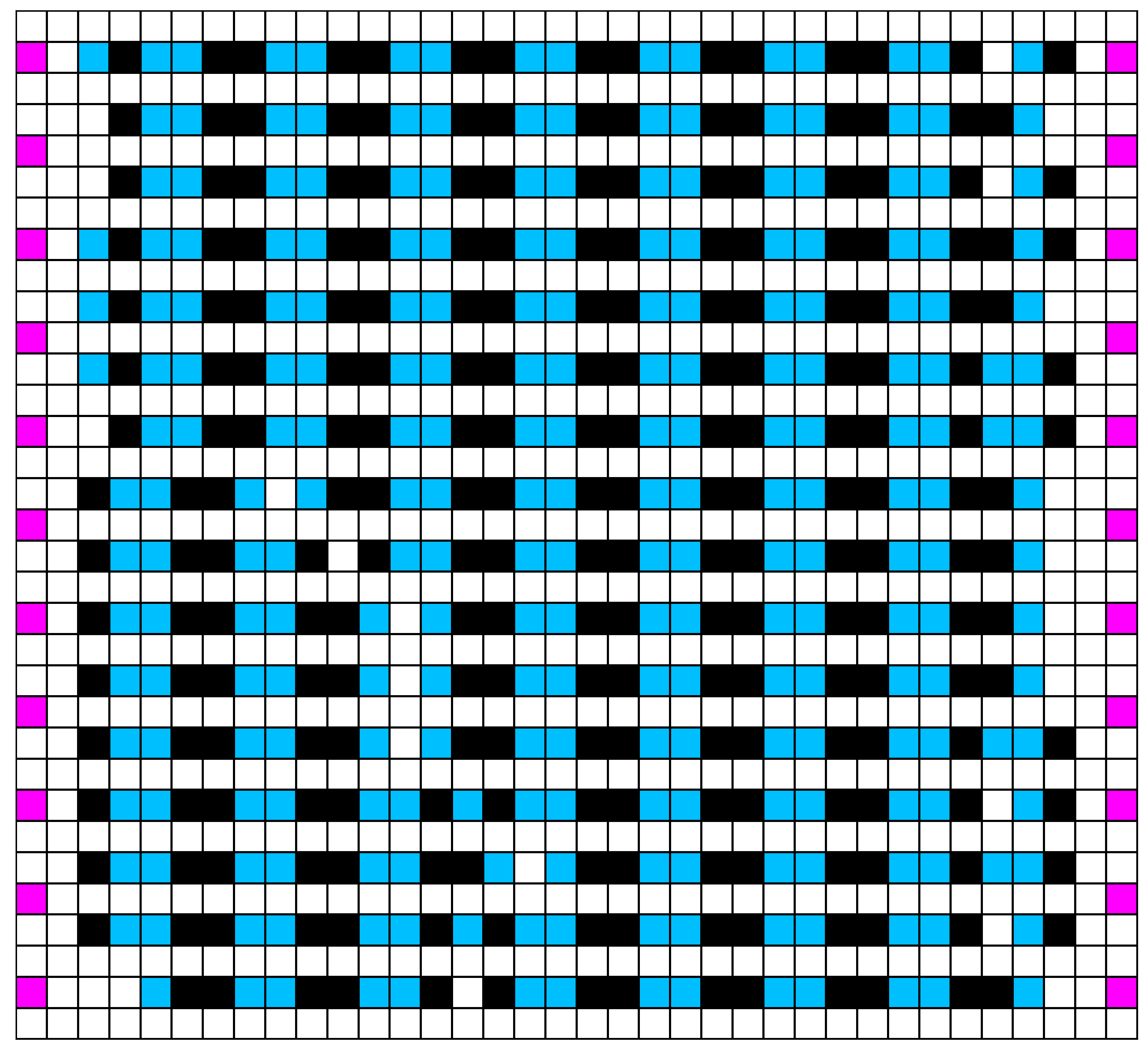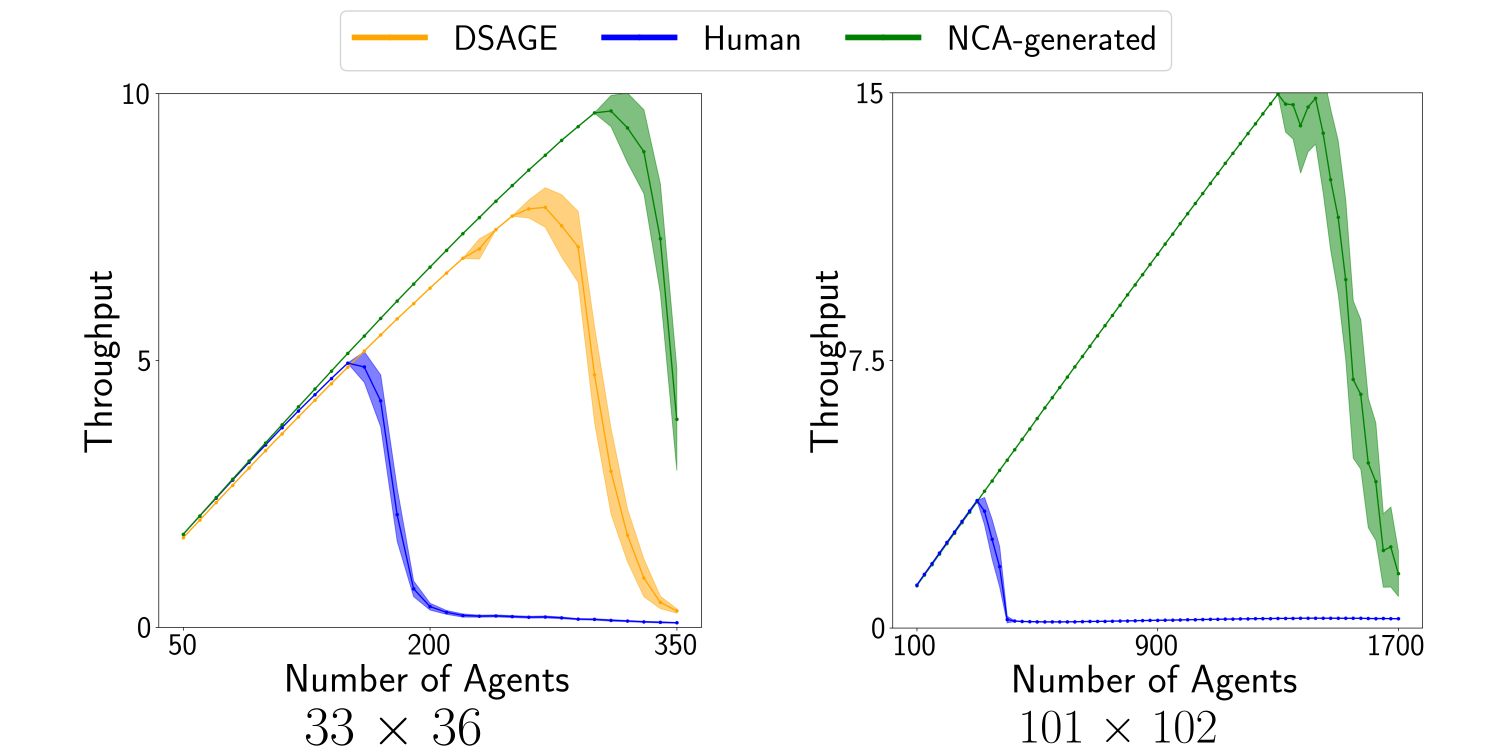# Arbitrarily Scalable Environment Generators via Neural Cellular Automata

### Abstract

We study the problem of generating arbitrarily large environments to improve the throughput of multi-robot systems. Prior work proposes Quality Diversity (QD) algorithms as an effective method for optimizing the environments of automated warehouses. However, these approaches optimize only relatively small environments, falling short when it comes to replicating real-world warehouse sizes. The challenge arises from the exponential increase in the search space as the environment size increases. Additionally, the previous methods have only been tested with up to 350 robots in simulations, while practical warehouses could host thousands of robots. In this paper, instead of optimizing environments, we propose to optimize Neural Cellular Automata (NCA) environment generators via QD algorithms. We train a collection of NCA generators with QD algorithms in small environments and then generate arbitrarily large environments from the generators at test time. We show that NCA environment generators maintain consistent, regularized patterns regardless of environment size, significantly enhancing the scalability of multi-robot systems in two different domains with up to 2,350 robots. Additionally, we demonstrate that our method scales a single-agent reinforcement learning policy to arbitrarily large environments with similar patterns.

Publication
In Proceedings of the Advances in Neural Information Processing Systems (NeurIPS), December 10–16, 2023, New Orleans, LA, USA

## Introduction

Our previous work  formulates the environment optimization problem as a Quality Diversity (QD) optimization problem and optimizes the environments by searching for combination of tiles. The optimized environments have much higher throughput and are more scalable than human-designed ones. However, with this method, the search space of the QD algorithm grows exponentially with the size of the environment.

Therefore, instead of optimizing the environments directly, this paper proposes to optimize Neural Cellular Automata (NCA) environment generators via QD algorithms. We show that NCA-generated environments maintain consistent, regularized patterns regardless of environment size, significantly enhancing the scalability of multi-robot systems.

## Approach Overview

We extend previous works [1,2] to use CMA-MAE  to search for a diverse collection of NCA generators with the objective and diversity measures computed from an agent-based simulator that runs domain-specific simulations in generated environments. Figure 1 provides an overview of our method. We start by sampling a batch of $b$ parameter vectors $\boldsymbol{\theta}$ from a multivariate Gaussian distribution, which form $b$ NCA generators. Each NCA generator then generates one environment from a fixed initial environment, resulting in $b$ environments. Figure 2 shows the architecture of the NCA generator.

We then repair the environments using a Mixed Integer Linear Programming (MILP) solver to enforce domain-specific constraints. After getting the repaired environments, we evaluate each of them by running an agent-based simulator for $N_e$ times, each with $T$ timesteps, and compute the average objective and measures. We add the evaluated environments and their corresponding NCA generators to both the optimization archive and the result archive. Finally, we update the parameters of the multivariate Gaussian distribution (i.e., $\mu$ and $\Sigma$) and sample a new batch of parameter vectors, starting a new iteration.Figure 1: Overview of our method.Figure 2: NCA generator architecture. It is a convolutional neural network (CNN) with 3 convolutional layers of kernel size 3 × 3 followed by ReLU or sigmoid activations.

## Results

### NCA-generated Environments

Figure 3 shows the NCA-generated environments of different sizes, showing that the trained NCA generators can generate environments of different sizes with consistent regularized patterns. Figure 4 shows an example NCA generation process of 200 iterations.33 $\times$ 36

### Scalability

We then analyze the scalability of the environments by running simulations with varying numbers of agents and show the throughput. We compare our NCA-generated environments with those optimized by DSAGE , the state-of-the-art environment optimization algorithm, and human-designed ones. Figure 5 shows the result. Our NCA-generated environments have higher throughput than the baseline environments with an increasingly larger gap with more agents.Figure 5: Throughput with an increasing number of agents in environments of size 33 $\times$ 36 and 101 $\times$ 102.

We further analyze the scalability of the NCA-generated environments in other sizes. Figure 6 shows the result. The y-axis illustrates two metrics: maximum mean throughput over 50 simulations (right) and the maximum scalability, defined as the agent count at this maximum (left).